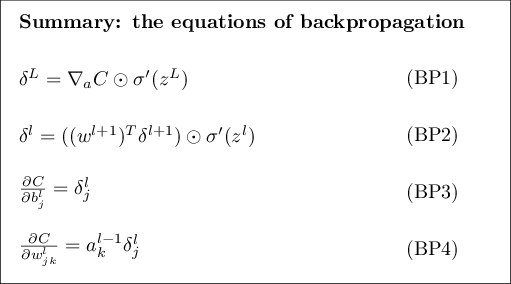Back to overviewNeural Net Problems - Exercise 6
April 14, 2020

Here are my solutions to exercise 6.

## The Backpropagation Algorithm

### Part 1 - Backpropagation with a Single Modified Neuron

#### Question

Suppose we modify a single neuron in a feedforward network so that the output from the neuron is given by , where is some function other than the sigmoid. How should we modify the backpropagation algorithm in this case?

#### Solution

We first would have to calculate the derviate for the function since it is needed for the backpropagation output error vector and . But other than that the neural network does not need any tweaking. You may think that it needs tweaking because is dependent on but we have defined and . We did this because it makes our lives easier for this particular case!

From Michael Nielsen:

You might wonder why the demon is changing the weighted input . Surely it'd be more natural to imagine the demon changing the output activation , with the result that we'd be using as our measure of error. In fact, if you do this things work out quite similarly to the discussion below. But it turns out to make the presentation of backpropagation a little more algebraically complicated. So we'll stick with as our measure of error.

### Part 2 - Backpropagation with Linear Neurons

#### Question

Suppose we replace the usual non-linear function with throughout the network. Rewrite the backpropagation algorithm for this case.

#### Solution

Since then , so it follows that . Also, .